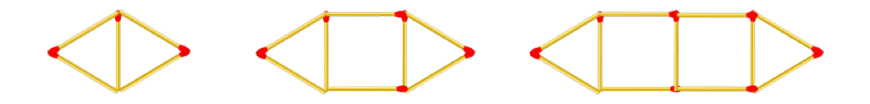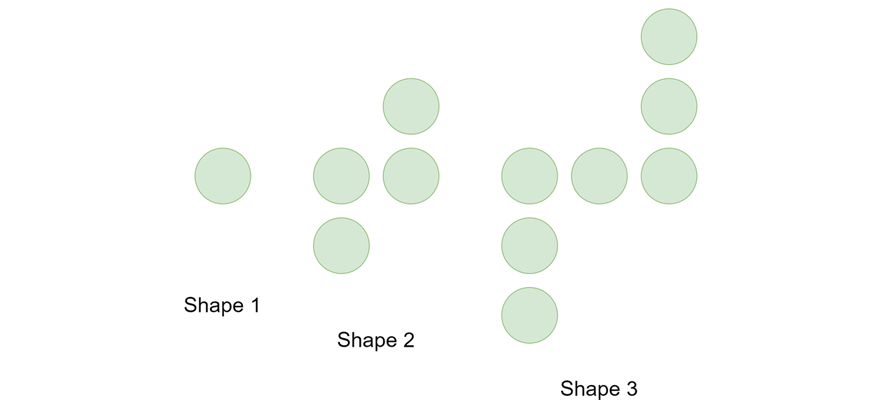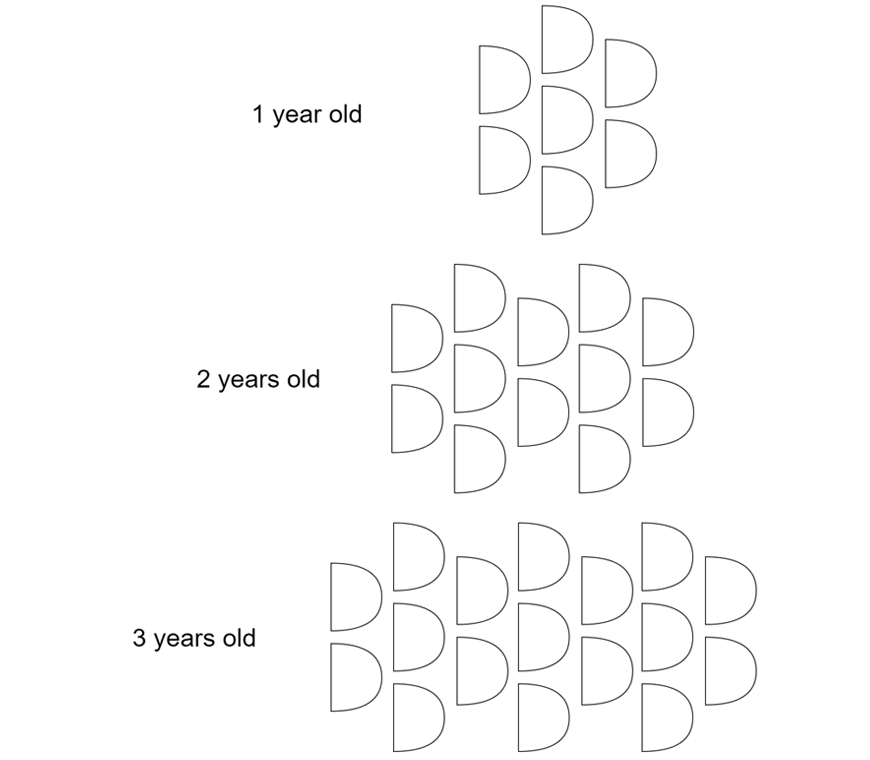# Part 2: Introduction to Linear Relationships

Previously, you would have needed to figure out the next numbers or shapes in a pattern. These are linear relationships. However, in Year 8, it becomes more challenging. In this guide, we will show you how you can use algebraic formulas to figure out the terms in a pattern!## Introduction to Linear Relationships

Many common mathematical relationships can be expressed as linear relationships.

For example, tax calculations or the relationship between speed, distance and time.

Studying them in Mathematics allows us to create and examine simple but effective models for relationships in the real world and make predictions and calculations.

## NSW Syllabus outcomes

SyllabusExplanation
Stage 4 NESA NSW Syllabus: ACMNA193
Use objects to build a geometric pattern, record the results in a table of values, describe the pattern in words and algebraic symbols​This means that you can use paddle pop sticks, match sticks or other objects to create patterns then, record the information into this table:

 Shape number $$(x)$$ $$1$$ $$2$$ $$3$$ Number of matchsticks $$(y)$$ $$3$$ $$5$$ $$7$$

Then, describe the pattern with words and algebraic symbols:

• The pattern is increasing by $$2$$.
• $$x + 2 = y$$
Check pattern descriptions by substituting further valuesThis means that you can sub $$x = 1, x = 2 \ and \ x = 3$$ to check if the pattern works.
Replace written statements describing patterns with equations written in algebraic symbolsThis means that you can change “the pattern is increasing by $$2$$” into “$$x + 2 = y$$“
Recognise a given number pattern (including decreasing patterns), complete a table of values, describe the pattern in words and algebraic symbolsThis means that you can figure out what the pattern is and do all of the above.
Use a rule generated from a pattern to calculate the corresponding value for a larger numberThis means that you can substitute $$x = 4$$ to find the next number in the sequence.

## Assumed knowledge

Students should be familiar with simple arithmetic techniques, such as substitution and using brackets, and basic algebra.

## Linear relationships

Consider the following matchstick pattern:For the first $$(1)$$ shape, there are $$5$$ matchsticks.

For the second $$(2)$$ shape, there are $$8$$ matchsticks.

For the third $$(3)$$ shape, there are $$11$$ matchsticks.

A pattern can be created that relates the shape number with the number of matchsticks used.

To do this, we can first draw a table of values:

 Shape number 1 2 3 Number of matchstick 5 8 11

Note: The difference between the first shape and the second shape is $$3$$ matchsticks, and the difference between the second shape and the third shape is also $$3$$ matchsticks.

Hence, we can write:

\begin{align*}
matchsticks \ = \ 3 \times \ shape \ number \ + \ constant
\end{align*}

In this case, when the shape number is $$1$$, we have $$5$$ matchsticks so the constant is $$(5-3) = 2$$.

This is an example of a linear relationship.

To be more concise, we can use an algebraic term instead of the number of matchsticks $$(m)$$ and another term for the shape number $$(s)$$:

\begin{align*}
m = 3s + 2
\end{align*}

To check this linear relationship, we can substitute $$s = 3$$ (the third shape):

\begin{align*}
m = 3 \times (3) + 2 = 11
\end{align*}

which matches the pattern we observed, confirming that the pattern works.

How many matches are in the $$12th$$ shape?

If $$s = 12$$, then:

\begin{align*}
m = 3 \times (12) + 2 = 28
\end{align*}

Thus, we can determine that the  $$12th$$ shape will have $$38$$ matchsticks.

Now imagine that you wanted to determine which shape number contains $$50$$ matchsticks.

In this case, you would substitute $$m = 50$$:

\begin{align*}
50 &= 3s + 2 \\
48 &= 3s \\
s &= \frac{48}{3} = 16
\end{align*}

Hence, $$50$$ matchsticks would generate the  $$16th$$ shape.

In conclusion, by using the above methods, you can determine the number of matchsticks needed for any shape in the pattern and the number that corresponds to a certain number of matchsticks.

## Summary of Introduction to Linear Relationships

When encountering a geometric sequence, first try to derive a pattern relating the figures together.

Creating a table of values may aid you in this process.

You can then check or use your pattern for larger numbers by substituting into the generated pattern.

## Boost your child’s Maths marks now!

Learn about linear relationships and other Year 8 Maths topics before it’s taught in school! Learn more about our Year 8 Maths Term Course.## Checkpoint questions

1) The number of blocks for a model depends on the number of levels:a. Create a pattern relating the number of blocks $$(b)$$ with the number of levels $$(l)$$.

b. How many blocks are required to build a model with $$15$$ levels?

c. If $$71$$ blocks were used to create a model, how many levels would it have?

2) A sequence of shapes is constructed using circles.a. Create a pattern relating the number of circles $$(c)$$ with the shape number $$(s)$$.

b. If I have $$30$$ circles, what is the largest shape I can build?

c. How many circles does the $$50th$$ shape have?

3) An armadillo grows scales each year in the following pattern:a. Determine the pattern relating the number of scales $$(s)$$ with the age of the armadillo $$(a)$$.

b. A scientist noticed an armadillo with $$57$$ scales. How old is it?

c. How many scales does an armadillo that is $$7$$ years old have?

## Solution:

1)

a. $$b = 2l + 1$$

b. $$31 \ blocks$$

c. $$35 \ levels$$

2)

a. $$c = 3s – 2$$

b. $$Shape \ 10$$

c. $$148 \ circles$$

3)

a. $$s = 5a + 2$$

b. $$11 \ years \ old$$

c. $$37$$

© Matrix Education and www.matrix.edu.au, 2021. Unauthorised use and/or duplication of this material without express and written permission from this site’s author and/or owner is strictly prohibited. Excerpts and links may be used, provided that full and clear credit is given to Matrix Education and www.matrix.edu.au with appropriate and specific direction to the original content.